拉格朗日对偶性_拉格朗日求最值对偶性 - CSDN

• 拉格朗日对偶性 参考：《统计学习方法》李航 约束优化问题中，常常利用拉格朗日对偶性将原始问题转换为对偶问题，通过解对对偶问题而得到原始问题。该方法应用在许多统计学习方法中，例如最大熵模型和支持向量机...
拉格朗日乘子法
摘自周志华《机器学习》
拉格朗日乘子法是一种寻找多元函数在一组约束下的极值的方法，通过引入拉格朗日乘子，可将有$d$个变量和$k$个约束条件的最优化问题转化为具有$d+k$个变量的无约束优化问题求解.
先考虑一个等式约束的优化问题，假定$\boldsymbol x$为$d$维向量，欲寻求$\boldsymbol x$的某个取值$\boldsymbol x^*$,使目标函数$f(\boldsymbol x)$最小且同时满足$g(\boldsymbol x)=0$的约束. 从几何角度来看，该问题的目标是在方程$g(\boldsymbol x)=0$确定的$d-1$维曲面上寻找能使目标函数$f(\boldsymbol x)$最小化的点. 由此可以得出如下结论:

对于约束曲面上的任意点$x$,该点的梯度$\nabla g(\boldsymbol{x})$正交于约束曲面;
在最优点$\boldsymbol x^*$，目标函数在该点的梯度$\nabla f(\boldsymbol{x^*})$正交于约束曲面.

由此可知，在最优点$\boldsymbol x^*$，如图1所示梯度$\nabla g(\boldsymbol{x})$与梯度$\nabla f(\boldsymbol{x})$的方向必相同或相反，即存在$\lambda \neq 0$使得$\nabla f\left(\boldsymbol{x}^{*}\right)+\lambda \nabla g\left(\boldsymbol{x}^{*}\right)=0 \quad(1)$ $\lambda$称为拉格朗日乘子 定义拉格朗日函数$L(\boldsymbol{x}, \lambda)=f(\boldsymbol{x})+\lambda g(\boldsymbol{x})\quad(2)$不难发现，将其对$x$的偏导数$\nabla_{\boldsymbol{x}} L(\boldsymbol{x}, \lambda)$置零得式$(1)$，同时，将其对$\lambda$的偏导数$\nabla_{\boldsymbol{\lambda}} L(\boldsymbol{x}, \lambda)$置零的得约束条件$g( \boldsymbol x)=0$，于是原约束优化问题可转化为对拉格朗日函数$L(\boldsymbol{x}, \lambda)$的无约束优化问题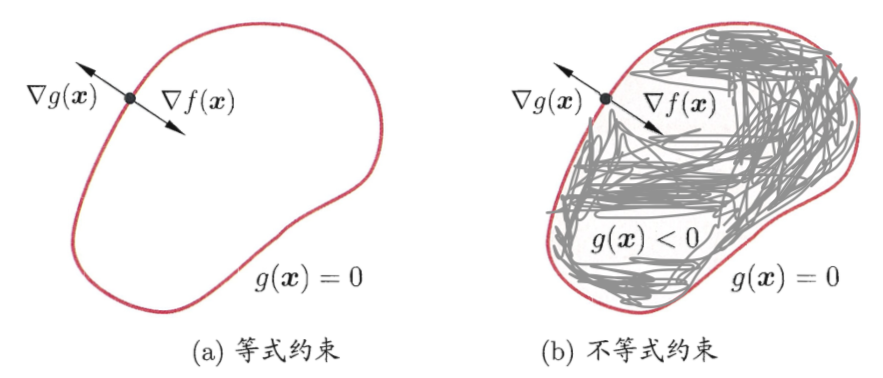图1  拉格朗日乘子法的几何含义：在$(a)$等式约束$g(\boldsymbol x)=0$或不等式约束$g(\boldsymbol{x}) \leqslant 0$下，最小化目标函数$f(x)$，红色曲线表示$g(\boldsymbol x)=0$构成的曲面，其围成的阴影区域表示$g(\boldsymbol{x}) < 0$

现考虑不等式约束$g(\boldsymbol{x}) \leqslant 0$，如图1所示，此时最优点$\boldsymbol x^*$或在$g(\boldsymbol{x}) < 0$的区域中，或在边界上$g(\boldsymbol x)=0$.

对于$g(\boldsymbol{x}) < 0$的情形，约束$g(\boldsymbol{x}) \leqslant 0$不起作用，可直接通过$\nabla f(\boldsymbol{x})=0$
来获取最优点；这等价于将$\lambda$置零然后对$\nabla_{\boldsymbol{x}} L(\boldsymbol{x}, \lambda)$置零得到最优点.
$g(\boldsymbol x)=0$的情形类似于上面等式约束的分析，需注意的是，此时$\nabla f(\boldsymbol{x^*})=0$的方向必与$\nabla g(\boldsymbol{x^*})=0$相反，即存在常数$\lambda >0$使得$\nabla f\left(\boldsymbol{x}^{*}\right)+\lambda \nabla g\left(\boldsymbol{x}^{*}\right)=0$.
整合这两种情形，必满足$\lambda g(\boldsymbol x)=0$因此在约束$g(\boldsymbol{x}) < 0$下最小化$f(\boldsymbol x)$，可转化为在约束下最小化式$(2)$的拉格朗日函数：
$\left\{\begin{array}{l}{g(\boldsymbol{x}) \leqslant 0} \\ {\lambda \geqslant 0} \qquad\qquad(3)\\ {\lambda g(\boldsymbol{x})=0}\end{array}\right.$式$(3)$称为$karush-Kuhn-Tucker$ (KKT)条件.
上述做法可推广到多个约束
$\min _{\boldsymbol{x}} f(\boldsymbol{x})$$\text { s.t. } \quad g_{j}(\boldsymbol{x}) \leqslant 0 \quad(j=1, \ldots, n) \qquad(4)$$h_{i}(\boldsymbol{x})=0 \quad(i=1, \ldots, m)$引入拉格朗日乘子$\boldsymbol{\lambda}=\left(\lambda_{1}, \lambda_{2}, \ldots, \lambda_{m}\right)^{\mathrm{T}}$和$\boldsymbol{\mu}=\left(\mu_{1}, \mu_{2}, \ldots, \mu_{n}\right)^{\mathrm{T}}$,相应的拉格朗日函数为
$L(\boldsymbol{x}, \boldsymbol{\lambda}, \boldsymbol{\mu})=f(\boldsymbol{x})+\sum_{j=1}^{n} \mu_{j} g_{j}(\boldsymbol{x})+\sum_{i=1}^{m} \lambda_{i} h_{i}(\boldsymbol{x}) \qquad(5)$由不等式约束引入的$KKT$由不等式约束引入的条件$(j=1,2, \dots, n)$为
$\left\{\begin{array}{l}{g_{j}(\boldsymbol{x}) \leqslant 0} \\ {\mu_{j} \geqslant 0}\qquad\qquad (6) \\ {\mu_{j} g_{j}(\boldsymbol{x})=0}\end{array}\right.$

拉格朗日对偶性
摘自李航《统计学习方法》
约束优化问题中，常常利用拉格朗日对偶性将原始问题转换为对偶问题，通过解对对偶问题而得到原始问题。该方法应用在许多统计学习方法中，例如最大熵模型和支持向量机。

1   原始问题

假设$f(x), c_{i}(x),h_{j}(x)$是定义在$R^n$上的连续可微函数。考虑约束最优化问题.
$\min _{x \in R^{n}} f(x) \qquad (1) \\ \text { s.t. } \quad c_{i}(x) \leqslant 0, \quad i=1,2, \cdots, k \qquad (2)\\ h_{j}(x)=0, \quad j=1,2, \cdots, l \qquad(3)$称此约束最优化问题为原始最优化问题或原始问题
首先，引进广义拉格朗日函数 ($generalized\, Lagrange \, function$)
$L(x, \alpha, \beta)=f(x)+\sum_{i=1}^{k} \alpha_{i} c_{i}(x)+\sum_{j=1}^{l} \beta_{j} h_{j}(x) \qquad (4) \\ x=\left(x^{(1)}, x^{(2)}, \cdots, x^{(n)}\right)^{\mathrm{T}} \in \mathbf{R}^{n},$ $\alpha_{i}, \beta_{j}$是拉格朗日乘子,$\alpha_{i} \geqslant 0$考虑$x$的函数$\theta_{P}(x)=\max _{\alpha, \beta ; \alpha_{i} \geqslant 0} L(x, \alpha, \beta) \qquad (5)$ 这里，下标$P$表示原始问题假设给定某个$x$，如果$x$违反原始问题的约束条件，即存在某个$i$，使得$c_i(x)>0$或者存在$j$某个使得$h_{j}(x) \neq 0$，那么就有$\theta_{P}(x)=\max _{\alpha, \beta : \alpha_{i} \geqslant 0}\left[f(x)+\sum_{i=1}^{k} \alpha_{i} c_{i}(x)+\sum_{j=1}^{l} \beta_{j} h_{j}(x)\right]=+\infty \qquad (6)$
因为若某个$i$使约束$c_i(x)>0$,则可令$\alpha_i \rightarrow+\infty$ ，若某个$j$使得，则可令使得$h_{j}(x) \neq 0$，而其余的$\alpha_{i}, \beta_{j}$,均取为0.相反地，如果$x$满足约束条件式$(2)$和式$(3)$，则由式$(5)$和式$(4)$可知$\theta_{P}(x)=f(x)$，因此，
$\theta_{P}(x)=\left\{\begin{array}{l}{f(x)} , x满足原始问题约束\\ {+\infty}，其他\end{array}\right. \quad(7)$
所以如果考虑极小化问题$\min _{x} \theta_{P}(x)=\min _{x} \max _{\alpha, \beta : \alpha_{i} \geqslant 0} L(x, \alpha, \beta) \qquad(8)$它是与原始优化问题$(1)\sim(3)$等价的，即它们有相同的解。问题
$\min _{x} \max _{\alpha, \beta : \alpha_{i} \geqslant 0} L(x, \alpha, \beta)$称为广义拉格朗日的极小极大问题，这样把原始问题表示为广义拉格朗日函数的极小极大问题。为了方便，定义原始问题的最优解$p^{*}=\min _{x} \theta_{P}(x) \qquad(9)$为原始问题的值。

2   对偶问题

定义$\theta_{D}(\alpha, \beta)=\min _{x} L(x, \alpha, \beta) \qquad(10)$再考虑极大化$\theta_{D}(\alpha, \beta)=\min _{x} L(x, \alpha, \beta)$，即$\max _{\alpha, \beta : \alpha_{i} \geq 0} \theta_{D}(\alpha, \beta)=\max _{\alpha, \beta ; \alpha_{i} \geqslant 0} \min _{x} L(x, \alpha, \beta) \qquad(11)$问题$\max _{\alpha, \beta ; \alpha_{i} \geqslant 0} \min _{x} L(x, \alpha, \beta)$称为广义拉格朗日函数的极大极小问题可以将广义拉格朗日的极大极小问题表示为约束最优化问题：$\max _{\alpha, \beta} \theta_{D}(\alpha, \beta)=\max _{\alpha, \beta} \min _{x} L(x, \alpha, \beta) \qquad(12)$$\text { s.t. } \quad \alpha_{i} \geqslant 0, \quad i=1,2, \cdots, k \qquad(13)$称为原始问题的对偶问题。
定义对偶问题的最优值$d^{*}=\max _{\alpha, \beta : \alpha_{i} \geqslant 0} \theta_{D}(\alpha, \beta) \qquad(14)$称为对偶问题的值。

3   原始问题和对偶问题的关系

定理1  $\;$若原始问题和对偶问题都有最优值，则
$d^{*}=\max _{\alpha, \beta : \alpha_{i} \geqslant 0} \min _{x} L(x, \alpha, \beta) \leqslant \min _{x} \max _{\alpha, \beta : \alpha_{i} \geqslant 0} L(x, \alpha, \beta)=p^{*} \qquad(15)$证明：由式$(12)$和$(5)$，对任意的$\alpha,\beta$和$x$，有
$\theta_{D}(\alpha, \beta)=\min _{x} L(x, \alpha, \beta) \leqslant L(x, \alpha, \beta) \leqslant \max _{\alpha, \beta : \alpha_{i} \geq 0} L(x, \alpha, \beta)=\theta_{P}(x) \quad(16)$即$\quad$$\theta_{D}(\alpha, \beta) \leqslant \theta_{P}(x) \qquad(17)$
由于原始问题和对偶问题均有最优解，所以，
$\max _{\alpha, \beta : \alpha_{i} \geqslant 0} \theta_{D}(\alpha, \beta) \leqslant \min _{x} \theta_{P}(x) \qquad(18)$
即$d^{*}=\max _{\alpha, \beta : \alpha_{i} \geqslant 0} \min _{x} L(x, \alpha, \beta) \leqslant \min _{x} \max _{\alpha, \beta : \alpha_{i} \geqslant 0} L(x, \alpha, \beta)=p^{*} \qquad(19)$
推论1$\;$ 设$x^*$ 和$\alpha^*,\beta^*$分别是原始问题$(1)\sim(3)$和对偶问题$(12)\sim(13)$的可行解，并且$d^*=p^*$,则$x^*$和$\alpha^*,\beta^*$分别是原始问题和对偶问题的最优解。
在某些条件下，原始问题和对偶问题的最优值相等，$d^*=p^*$，这时可以用解对偶问题代替原始问题。
定理2:$\;$ 考虑原始问题$(1)\sim(3)$和对偶问题$(12)\sim(13)$。假设函数$f(x)$和$c_i(x)$是凸函数，$h_j(x)$是仿射函数；并且假设不等式约束$c_i(x)$是严格可行的，即存在$x$，对所有$i$有$c_i(x)<0$，并且存在$x^{*}, \alpha^{*}, \beta^{*}$，使$x^*$是原始问题的解，$\alpha^{*}, \beta^{*}$是对偶问题的解，并且$p^{*}=d^{*}=L\left(x^{*}, \alpha^{*}, \beta^{*}\right) \qquad(20)$定理3:$\;$对原始问题$(1)\sim(3)$和对偶问题$(12)\sim(13)$。假设函数$f(x)$和$c_i(x)$是凸函数，$h_j(x)$是仿射函数；并且假设不等式约束$c_i(x)$是严格可行的，即存在$x$，对所有$i$有$c_i(x)<0$，并且存在$x^{*}, \alpha^{*}, \beta^{*}$，使$x^*$是原始问题的解，$\alpha^{*}, \beta^{*}$是对偶问题的解充分必要条件是$x^{*}, \alpha^{*}, \beta^{*}$满足下面的$Karush-Kuhn-Tucker(KKT)$条件：$\nabla_{x} L\left(x^{*}, \alpha^{*}, \beta^{*}\right)=0 \qquad(21)$$\nabla_{\alpha} L\left(x^{*}, \alpha^{*}, \beta^{*}\right)=0 \qquad(22)$$\nabla_{\beta} L\left(x^{*}, \alpha^{*}, \beta^{*}\right)=0 \qquad(23)$$\alpha_{i}^{*} c_{i}\left(x^{*}\right)=0, \quad i=1,2, \cdots, k \quad(24)$$c_{i}\left(x^{*}\right) \leqslant 0, \quad i=1,2, \cdots, k \qquad(25)$$\alpha_{i}^{*} \geqslant 0, \quad i=1,2, \cdots, k \qquad(26)$$h_{j}\left(x^{*}\right)=0 \quad j=1,2, \cdots, l \qquad(27)$特别指出，式$(24)$称为KKT的对偶互补条件，由此条件可知：若$\alpha_{i}^{*}>0$，则$c_{i}\left(x^{*}\right)=0$


展开全文•   在约束最优化问题中，常常利用拉格朗日对偶性将原始问题转换为对偶问题，通过解对偶问题而得到原始问题的解。该方法应用在许多统计学习方法中，例如，最大熵模型与支持向量机。 1、原始问题   假设f(x)f(x)f...
  在约束最优化问题中，常常利用拉格朗日对偶性将原始问题转换为对偶问题，通过解对偶问题而得到原始问题的解。该方法应用在许多统计学习方法中，例如，最大熵模型与支持向量机。
1、原始问题
假设$f(x)$,$c_{i}(x)$,$h_{j}(x)$是定义在$R^n$上的连续可微函数。考虑约束最优化问题
$\min_{x\in R^n} f(x) \tag{C.1}$
$s.t. \ c_{i}(x)\le 0，i = 1,2,\ldots,k \tag{C.2}$
$h_{j}(x)=0，j=1,2,\ldots,l \tag{C.3}$
称此约束最优化问题为原始最优化问题或原始问题。
首先，引入广义拉格朗日函数
$L(x,\alpha,\beta) = f(x)+\sum_{i=1}^{k}\alpha_{i}c_{i}(x) + \sum_{j=1}^l \beta_{j}h_{j}(x) \tag{C.4}$
这里，$x=(x^{(1)},x^{(2)},\ldots,x^{(n)}) \in R^{n}$,$\alpha_{i},\beta_{j}$是拉格朗日乘子，$\alpha_{i} \ge 0$。考虑$x$的函数：
$\theta_{p}(x) = \max_{\alpha,\beta:\alpha_{i}\ge 0} L(x,\alpha,\beta) \tag{C.5}$
这里，下标$P$表示原始问题。
假设给定某个x,如果x违反原始问题的约束条件，即存在某个i使得$c_{i}(w) \gt 0$或者存在某个j使得$h_{j}(w) \ne 0$,那么有.
$\theta_{p}{x}=\max_{\alpha,\beta:\alpha_{i}\ge 0}[f(x)+\sum_{i=1}^{k}\alpha_{i}c_{i}(x) + \sum_{j=1}^l \beta_{j}h_{j}(x)] = +\infty \tag{C.6}$
因为若某个i使约束$c_{i}(x) \gt 0$,则可令$\alpha_{i} \rightarrow +\infty$,若某个$j$使$h_{j}(x) \ne 0$,则可令$\beta_{j}$使得$\beta_{j}h_{j}(x)\rightarrow +\infty$,而将其余各$\alpha_{i},\beta_{j}$均取为0。
相反地，如果$x$满足约束条件式(C.2)和(C.3),则由式(C.5)和式(C.4)可知，$\theta_{p}(x)=f(x)$。因此，
$\theta_{p}(x)=\begin{cases} f(x)，&x满足原始条件约束\\ +\infty，&其他 \end{cases} \tag{C.7}$
所以如果考虑极小化问题
$\min_{x}\theta_{p}(x)=\min_{x}\max_{\alpha,\beta:\alpha_{i}\ge 0}L(x,\alpha,\beta) \tag{C.8}$
它是与原始最优化问题(C.1~C.3)等价的，即他们由相同的解。问题$\min \limits_{x}\max \limits_{\alpha,\beta:\alpha_{i}\ge 0}L(x,\alpha,\beta)$称为广义拉格朗日极小极大问题。这样，就把原始问题的最优值
$p^*=\min_{x}\theta_{p}(x) \tag{C.9}$
称为原始问题的值。
2、对偶问题
定义
$\theta_{D}(\alpha,\beta) = \min_{x}L(x,\alpha,\beta) \tag{C.10}$
在考虑极大化$\theta_{D}(\alpha,\beta) = \min_{x}L(x,\alpha,\beta)$，即
$\max_{\alpha,\beta:\alpha_{i}\ge 0}\theta_{D}(\alpha,\beta)=\max_{\alpha,\beta:\alpha_{i}\ge 0}\min_{x}L(x,\alpha,\beta) \tag{C.11}$
问题$\max \limits_{\alpha,\beta:\alpha_{i}\ge 0}\min_{x}L(x,\alpha,\beta)$称为广义拉格朗日函数的极大极小问题。
可以将广义拉格朗日函数的极大极小问题表示为约束最优化问题：
$\max_{\alpha,\beta}\theta_{D}(\alpha,\beta)=\max_{\alpha,\beta}\min_{x}L(x,\alpha,\beta) \tag{C.12}$
$s.t. \ \alpha_{i}\ge 0，i=1,2,\ldots,k \tag{C.13}$
称为原始问题的对偶问题。定义对偶问题的最优值
$d^*=\max_{\alpha,\beta:\alpha_{i}\ge 0}\theta_{D}(\alpha,\beta) \tag{C.14}$
称为对偶问题的值。
3、原始问题和对偶问题的关系
定理C.1 若原始问题对偶问题都有最优值，则
$d^*=\max_{\alpha,\beta:\alpha_{i}\ge 0}\min_{x}L(x,\alpha,\beta) \le \min_{x}\max_{\alpha,\beta:\alpha_{i}\ge 0}L(x,\alpha,\beta) = p^* \tag{C.15}$
证明 由式$(C.12)$和式$(C.5)$,对任意的$\alpha,\beta$和$x$,有
$\theta_{D}(\alpha,\beta) = \min_{x}L(x,\alpha,\beta) \le L(x,\alpha,\beta) \le \max_{\alpha,\beta:\alpha_{i}\ge 0}L(x,\alpha,\beta) = \theta_{p}(x) \tag{C.16}$
即
$\theta_{D}(\alpha,\beta) \le \theta_{p}(x) \tag{C.17}$
由于原始问题和对偶问题均有最优值，所以，
$\max_{\alpha,\beta:\alpha_{i}\ge 0}\theta_{D}(\alpha,\beta) \le \min_{x} \theta_{p}(x) \tag{C.18}$
即
$d^*=\max_{\alpha,\beta:\alpha_{i}\ge 0}\min_{x}L(x,\alpha,\beta) \le \min_{x}\max_{\alpha,\beta:\alpha_{i}\ge 0}L(x,\alpha,\beta) = p^* \tag{C.19}$
推论C.1  设$x^*$和$\alpha^*,\beta^*$分别为原始问题(C.1)-(C.3)和对偶问题(C.12)-(C.13)的可行解，并且$d^*=p^*$,则$x^*$和$\alpha^*,\beta^*$分别式原始问题和对偶问题的最优解。
定理C.2 考虑原始问题(C.1)-(C.3)和对偶问题(C.12)-(C.13)。假设函数$f(x)$和$c_{i}(x)$是凸函数，$h_{j}(x)$是仿射函数；并且假设不等式约束$c_{i}(x)$是严格可行的，即存在x，对所与$i$有$c_{i}(x) \lt 0$,则存在$x^*,\alpha^*,\beta^*$,使$x^*$是原始问题的解，$\alpha^*$,$\beta^*$是对偶问题的解，并且\$
$p^*=d^*=L(x^*,\alpha^*,\beta^*) \tag{C.20}$
***定理C.3***对原始问题(C.1)-(C.3)和对偶问题(C.12)-(C.13)，假设函数$f(x)$和$c_{i}(x)$是凸函数，$h_{j}(x)$是仿射函数，并且假设不等式约束$c_{i}(x)$是严格可行的则$x^*$和$\alpha^*,\beta^*$分别式原始问题和对偶问题的解的充分必要条件是$x^*,\alpha^*,\beta^*$满足下面的Karush-Kuhn-Tucker(KKT)条件：
$\nabla_{x}L(x^*,\alpha^*,\beta^*)=0 \tag{C.21}$
$\alpha^*c_{i}(x^*)=0,\ i=1,2,\ldots,k \tag{C.22}$
$c_{i}(x^*) \le 0, \ i=1,2,\ldots,k \tag{C.23}$
$\alpha^* \ge 0, \ i=1,2,\ldots,k \tag{C.24}$
$h_{j}(x^*) = 0,\ j = 1,2,\ldots,l \tag{C.25}$
特别指出，式(C.24)称为KKT的队友互补条件。由此条件可知：若$\alpha^* \gt 0$，则$c_{i}(x^*) = 0$


展开全文• 在支持向量机和最大熵模型中都会用到拉格朗日对偶性，主要为解决约束最优化问题，通过将原始问题转换为对偶问题求解。为方便理解，遂记录下简单的概念的结论，有理解不当的地方望多提意见~ 1. 原始问题 先从最...
引言

在支持向量机和最大熵模型中都会用到拉格朗日对偶性，主要为解决约束最优化问题，通过将原始问题转换为对偶问题求解。为方便理解，遂记录下简单的概念的结论，有理解不当的地方望多提意见~

1. 原始问题

先从最简单的求函数最小值开始说起：

minx∈Rnf(x)\min_{\boldsymbol{x} \in \mathbf{R}^n}f(\boldsymbol{x})

求f(x)$f(x)$的最小值时x$x$的取值，f(x)$f(x)$在Rn$R^n$上连续可微。这时候我们对f(x)$f(x)$求导令导数为0就能取到极值了。若此时加入约束如下：

minx∈Rnf(x)\min_{\boldsymbol{x} \in \mathbf{R}^n}f(\boldsymbol{x})

其中f(x),ci(x),hj(x)在Rn$f(x),c_i(x),h_j(x)在R^n$上连续可微我们称此约束最优化为原始问题，也是拉格朗日对偶性需要解决的问题。
此时我们直接求导是无法解决了，要是可以将约束条件转换为未知变量或许就可以找到答案了。

2. 拉格朗日函数

为了求解原始问题，我们首先引入广义拉格朗日函数(generalized Lagrange function)：
L(x,α,β)=f(x)+∑i=1kαici(x)+∑j=1lβjhj(x)L(\boldsymbol{x},\boldsymbol{\alpha},\boldsymbol{\beta})=f(\boldsymbol{x})+\sum_{i=1}^k \alpha_ic_i(\boldsymbol{x}) + \sum_{j=1}^l\beta_jh_j(\boldsymbol{x})
其中x=(x1,x2,⋯,xn)T∈Rn$\boldsymbol{x}=(x_1,x_2,\cdots,x_n)^T \in \mathbf{R}^n$，αi和βj$\alpha_i和\beta _j$是拉格朗日乘子且αi≥0$\alpha_i\ge 0$
拉格朗日函数虽然一眼看去十分复杂，但是其实它是将所有的限定条件加上新引入的变量(拉格朗日乘子)构成了一个新的函数，这样就将限定条件转换为了未知变量。
此时我们考虑 x$x$ 的函数：
θP(x)=maxα,β:αi≥0L(x,α,β)\theta_P(\boldsymbol{x})=\max_{\boldsymbol{\alpha,\beta}:\alpha_i\ge0}L(\boldsymbol{x},\boldsymbol{\alpha},\boldsymbol{\beta})
下标P$P$代表原始问题。
可以证明，如果 x$x$满足原始问题中约束，那么θ(x)=f(x)$\theta(\boldsymbol{x})=f(\boldsymbol{x})$如果 x不满足原始问题中的约束(ci(x)≤0;hj(x)=0$c_i(x)\le 0;h_j(x)=0$)，那么θ(x)=+∞$\theta(\boldsymbol{x})=+\infty$，即：
θP(x)={f(x),+∞,x满足原始问题约束其他% <![CDATA[
\theta_P(\boldsymbol{x})=\begin{cases}f(\boldsymbol{x}),&\boldsymbol{x} 满足原始问题约束\\+\infty,&其他\end{cases} %]]>

证明：假设某个x$x$不满足原始问题的约束条件，即存在某个i$i$使得ci(x)>0$c_i(\boldsymbol{x})\gt0$或者存在某个j$j$使得hj(x)=0$h_j(\boldsymbol{x})=0$那么就有：
θP(x)=maxα,β:αi≥0[f(x)+∑i=1kαici(x)+∑j=1lβjhj(x)]=+∞\theta_P(\boldsymbol{x})=\max_{\boldsymbol{\alpha,\beta}:\alpha_i\ge0}\Bigg[f(\boldsymbol{x})+\sum_{i=1}^k \alpha_ic_i(\boldsymbol{x}) + \sum_{j=1}^l\beta_jh_j(\boldsymbol{x})\Bigg]=+\infty
因为若某个i$i$使约束ci(x)>0$c_i(\boldsymbol{x})\gt0$，则可令αi→+∞$\alpha_i\rightarrow+\infty$；
若某个j$j$使hj(x)≠0$h_j(\boldsymbol{x})\neq0$，则可令βj$\beta_j$使βjhj(x)→+∞$\beta_jh_j(\boldsymbol{x})\rightarrow+\infty$,而将其余各αi,βj$\alpha_i,\beta_j$均取为 0

所以如果考虑极小化问题：
minxθP(x)=minxmaxα,β:αi≥0L(x,α,β)\min_{\boldsymbol{x}}\theta_P(\boldsymbol{x})=\min_{\boldsymbol{x}}\max_{\boldsymbol{\alpha,\beta}:\alpha_i\ge0}L(\boldsymbol{x},\boldsymbol{\alpha},\boldsymbol{\beta})
就会发现它是与原始最优化问题等价的，即它们有相同的解。minxmaxα,β:αi≥0L(x,α,β)$\min_{\boldsymbol{x}}\max_{\boldsymbol{\alpha,\beta}:\alpha_i\ge0}L(\boldsymbol{x},\boldsymbol{\alpha},\boldsymbol{\beta})$称为广义拉格朗日函数的极小极大问题。这样一来，就把原始最优化问题表示为广义拉格朗日函数的极小极大问题。我们定义原始问题最优解：
p∗=minxθP(x)p^*=\min_{x}\theta_P(x)
总结一下原始问题和拉格朗日函数：从原始问题开始，通过拉格朗日函数重新定义一个无约束问题，这个无约束问题等价于原来的约束优化问题，从而将约束问题无约束化。也就是将d个变量和k个约束条件的最优化问题转换为d+k个变量的最优化问题。到此我们还是无法求解，我们需要将原始问题转换成对偶问题来求解。

3. 对偶问题

我们再定义：
θD(α,β)=minxL(x,α,β)\theta_D(\alpha,\beta)=\min_x L(x,\alpha,\beta)
并极大化θD$\theta_D$，即：
maxα,βθD(α,β)=maxα,βminxL(x,α,β)\max_{\alpha,\beta}\theta_D(\alpha,\beta)=\max_{\boldsymbol{\alpha,\beta}}\min_{\boldsymbol{x}}L(\boldsymbol{x},\boldsymbol{\alpha},\boldsymbol{\beta})
maxα,βminxL(x,α,β)$\max_{\boldsymbol{\alpha,\beta}}\min_{\boldsymbol{x}}L(\boldsymbol{x},\boldsymbol{\alpha},\boldsymbol{\beta})$为广义拉格朗日函数的极大极小问题(上一节的是极小极大问题)。这样可以将广义拉格朗日函数的极大极小问题进一步表示为约束最优化问题：
我们将上面的问题称为原始问题的对偶问题。定义对偶问题的最优值
d∗=maxα,βθD(α,β)d^* = \max_{\alpha,\beta}\theta_D(\alpha,\beta)
对比原始问题，对偶问题是先固定α,β$\alpha,\beta$，求最优化x$x$的解，在确定参数α,β$\alpha,\beta$；
原始问题是先固定x$x$，求最优化α,β$\alpha,\beta$的解，再确定x$x$。

4. 原始问题和对偶问题的关系

若原始问题和对偶问题都有最优值，那么
d∗=maxα,βminxL(x,α,β)≤minxmaxα,β:αi≥0L(x,α,β)=p∗d^*=\max_{\boldsymbol{\alpha,\beta}}\min_{\boldsymbol{x}}L(\boldsymbol{x},\boldsymbol{\alpha},\boldsymbol{\beta})\le\min_{\boldsymbol{x}}\max_{\boldsymbol{\alpha,\beta}:\alpha_i\ge0}L(\boldsymbol{x},\boldsymbol{\alpha},\boldsymbol{\beta})=p^*

证明:
对任意的x,α,β$x,\alpha,\beta$有
minxL(x,α,β)≤L(x,α,β)≤maxα,β:αi≥0L(x,α,β)\min_{\boldsymbol{x}}L(\boldsymbol{x},\boldsymbol{\alpha},\boldsymbol{\beta})\le L(\boldsymbol{x},\boldsymbol{\alpha},\boldsymbol{\beta})\le \max_{\boldsymbol{\alpha,\beta}:\alpha_i\ge0}L(\boldsymbol{x},\boldsymbol{\alpha},\boldsymbol{\beta})
由于原始问题和对偶问题均有最优值，所以：
minxL(x,α,β)≤maxα,β:αi≥0L(x,α,β)\min_{\boldsymbol{x}}L(\boldsymbol{x},\boldsymbol{\alpha},\boldsymbol{\beta})\le \max_{\boldsymbol{\alpha,\beta}:\alpha_i\ge0}L(\boldsymbol{x},\boldsymbol{\alpha},\boldsymbol{\beta})
即
d∗=maxα,βminxL(x,α,β)≤minxmaxα,β:αi≥0L(x,α,β)=p∗d^*=\max_{\boldsymbol{\alpha,\beta}}\min_{\boldsymbol{x}}L(\boldsymbol{x},\boldsymbol{\alpha},\boldsymbol{\beta})\le\min_{\boldsymbol{x}}\max_{\boldsymbol{\alpha,\beta}:\alpha_i\ge0}L(\boldsymbol{x},\boldsymbol{\alpha},\boldsymbol{\beta})=p^*

也就是说原始问题的最优值不小于对偶问题的最优值，但是我们要通过对偶问题来求解原始问题，就必须使得原始问题的最优值与对偶问题的最优值相等，于是可以得出下面的推论：

设x∗和α∗,β∗$x^*和\alpha^*,\beta^*$分别是原始问题和对偶问题的可行解并且d∗=p∗$d^*=p^*$则x∗和α∗,β∗$x^*和\alpha^*,\beta^*$分别是原始问题和对偶问题的最优解。

对偶问题跟原始问题可以看成本来是两个问题，因为优化的顺序不同而会得出两个不一定相关的值（但是 minxmaxyf(x,y)≥maxymaxxf(x,y)$\mathop{min}_{x}\mathop{max}_{y}f(x,y) \geq \mathop{max}_{y}\mathop{max}_{x}f(x,y)$ 还是成立的，直观理解的话高中经常用的二次函数就可以了）。
两者的差值就是duality gap，描述了我用另一种方式刻画问题的时候所造成的误差，强对偶的情况下最优值没有差别。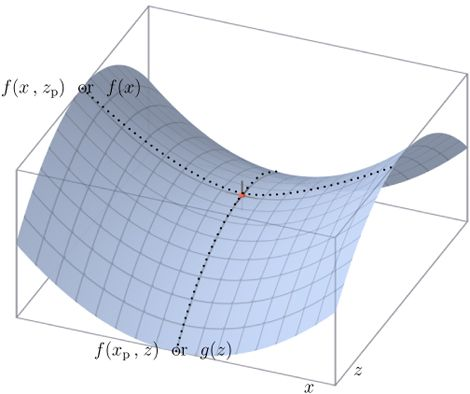在最优点处将会满足KKT 条件，但是KKT条件本身并不需要问题满足强对偶。关于KKT条件什么时候不满足，有一种另外的理解是他要求各个函数的梯度张成足够大的空间（因为KKT的最后一条本质上是一个Ax=0的问题）。
所以，当原始问题和对偶问题的最优值相等：d∗=p∗$d^*=p^*$时，可以用求解对偶问题来求解原始问题（当然是对偶问题求解比直接求解原始问题简单的情况下），但是到底满足什么样的条件才能使d∗=p∗$d^*=p^*$呢，这就是下面要阐述的 KKT 条件。

5. KTT条件

对原始问题和对偶问题，假设函数f(x)和ci(x)$f(x)和c_i(x)$是凸函数,hj(x)$h_j(x)$为仿射函数，并且不等式约束 ci(x)$c_i(x)$是严格可行的，则x∗和α∗,β∗$x^*和\alpha^*,\beta^*$分别是原始问题和对偶问题的解的充要条件是x∗,α∗,β∗$x^*,\alpha^*,\beta^*$满足下面的Karush-Kuhn-Tucker(KKT)条件:

∇xL(x∗,α∗,β∗)=0$\nabla_\boldsymbol{x}L(\boldsymbol{x}^*,\boldsymbol{\alpha}^*,\boldsymbol{\beta}^*)=0$
∇αL(x∗,α∗,β∗)=0$\nabla_\boldsymbol{\alpha}L(\boldsymbol{x}^*,\boldsymbol{\alpha}^*,\boldsymbol{\beta}^*)=0$
∇βL(x∗,α∗,β∗)=0$\nabla_\boldsymbol{\beta}L(\boldsymbol{x}^*,\boldsymbol{\alpha}^*,\boldsymbol{\beta}^*)=0$
α∗ici(x∗)=0,i=1,2,⋯,k$\alpha_i^*c_i(\boldsymbol{x}^*)=0,\quad i=1,2,\cdots,k$
ci(x∗)≤0,i=1,2,⋯,k$c_i(\boldsymbol{x}^*)\le0,\quad i=1,2,\cdots,k$
α∗i≥0,i=1,2,⋯,k$\alpha_i^*\ge0,\quad i=1,2,\cdots,k$
hj(x∗)=0,j=1,2,⋯,l$h_j(\boldsymbol{x}^*)=0,\quad j=1,2,\cdots,l$

关于KKT 条件的理解：前面三个条件是对于各个变量的偏导数为0（这就解释了一开始为什么假设三个函数连续可微，如果不连续可微的话，这里的偏导数存不存在就不能保证），后面四个条件就是原始问题的约束条件以及拉格朗日乘子需要满足的约束。

仿射函数
f(x)=Ax+bf(\boldsymbol{x})=\boldsymbol{A}\boldsymbol{x}+b
仿射函数就是一个线性函数，其输入是n维向量，参数 A可以是常数，也可以是m×n的矩阵，b可以是常数，也可以是m维的列向量，输出是一个m维的列向量。在几何上，仿射函数是一个线性空间到另一个线性空间的变换。

6. 总结

拉格朗日对偶性的求解分为两个步骤：

把原始的约束问题通过拉格朗日函数转化为无约束问题。
在满足KKT的条件下用求解对偶问题来代替求解原始问题，使得问题求解更加容易。

参考文献

李航，统计学习方法
周志华，机器学习（西瓜书）
https://www.cnblogs.com/90zeng/p/Lagrange_duality.html
https://www.zhihu.com/question/58584814
https://zhuanlan.zhihu.com/p/26514613

展开全文拉格朗日对偶性 优化
• 本文主要介绍拉格朗日乘子法到KKT条件的论证，再到拉格朗日对偶性的证明。


可行解和最优解
可行解：各种规划中画不等式组表示的平面区域（即是可行域）后该区域中的点都算可行解
最优解：通过几何方法在这个区域中可以找出约束条件的最值即最优解

拉格朗日乘子法
待求解问题为：
$min f(x)$
$s.t. \quad h_i(x) =0 \quad i=1,2,3,...,n$
这个问题可以转换为
$min [f(x)+\sum_{i=1}^{n} \alpha_i h_i(x)]$
其中$\alpha _i$称为拉格朗日乘子，
求解过程如下，
首先对拉格朗日方程$L(x, \alpha)=f(x)+\sum_{i=1}^{n} \alpha_i h_i(x)$的$x$和$\alpha$求导，得到如下式子，
$\left\{\begin{matrix} \nabla_x L(x,\alpha )=0\\ \nabla_\alpha L(x,\alpha ) =0 \end{matrix}\right.$
和 $h_i(x)$联立可得到最优解的$x$和$\alpha$,
下面证明算法的正确性，
现在有一个二元函数，要求其最小值，$min(f(x,y))$,而约束条件为$g(x,y)=c$, 则如下图所示，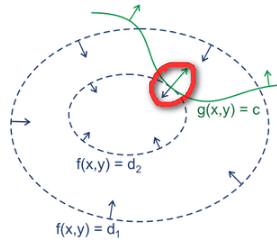通过图上可以看出最优解发生在$g(x,y)=c$的负梯度方向和$f(x,y)$某一等值线的梯度方向相同，即
$\nabla f(x,y) = -\alpha\nabla (g(x,y)-c) \rightarrow \nabla [f(x,y)+\alpha (g(x,y)-c)]=0$
通过上述推导，可得$L(x,y,\alpha)=f(x,y)+\alpha (g(x,y)-c)$的偏导等于0， 则说明这时分别对x,y,$\alpha$求偏导，可得
$\nabla_{x,y,\alpha }L(x,y,\alpha )=0$
$g(x,y)=c$
因而当$L(x,y,\alpha)$达到极值时和$f(x,y)$相同，因而达到极值时$g(x,y)-c=0$,这就证明了两个问题是等价的，

KKT条件
更一般的情况是求解约束条件下目标函数的极值问题既有等式，也有不等式，如下讲解只有不等式约束的情况，等式约束直接添加即可，
重申问题，
$min \quad f(x)$
$s.t. \quad g(x) \leq 0$
对应的拉格朗日公式为$L(x,\alpha)=f(x)+\alpha g(x)$， 图形表示形式如下，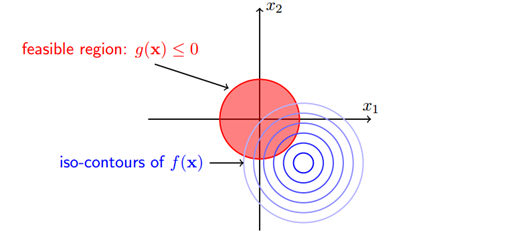这时，有两种情况，

如果可行解直接落在约束条件范围内，即落在$g(x)<0$的范围内，则直接删掉约束条件即可，
如果可行解落在约束条件外，则最优解在边界上去的，即在$g(x)=0$的曲线上取得，

上述两种状况如下所示，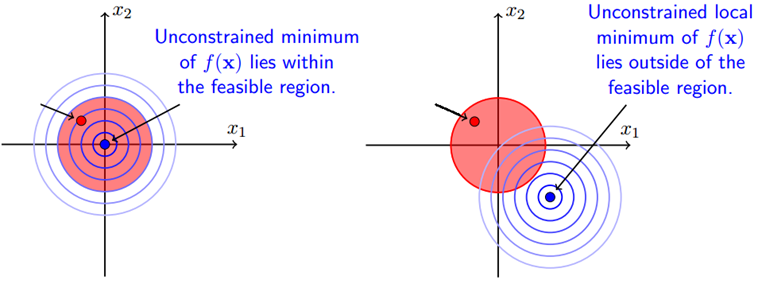以上两种状况要么落在约束区域内，则$\alpha=0$，因为直接去掉约束条件即可，要么落在约束条件边界上，则$g(x)=0$, 综合起来就是
$\alpha g(x)=0$
还有一个问题就是$\alpha$的取值问题，当$\alpha$不等于0的时候，即最优解在$g(x)=0$上取得时，$f(x,y)$的等值线的负梯度方向必须要和$g(x)=0$的梯度方向(法线方向)一致，即
$-\nabla_x f(x)= \alpha \nabla_x g(x)$
通过上面式子看出当$\alpha$不等于0的时候，$\alpha$一定大于0， 即$\alpha \geq 0$
只有这样才能够得到最优解，其他虽然共线但是方向不同的不算是最优解，解释如下图所示，、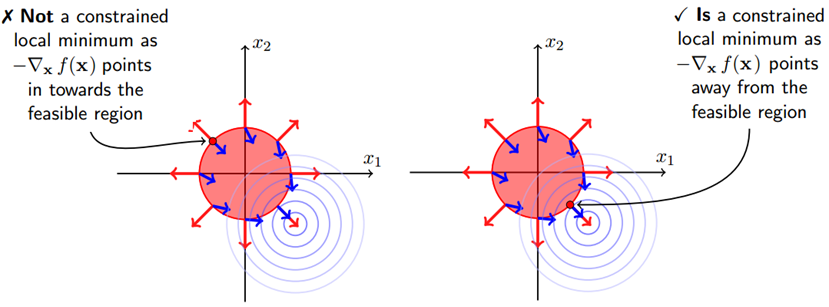因而对于不等式约束，只要满足一定的条件都能够用拉格朗日算子法求解，这里的条件就是所谓的KKT条件，条件的总和即为上面讲述的结果，
整理一下可得，目标函数为
$min(f(x))$
$s.t. \quad h_i(x)=0, \quad i=1,2,...,m$
$\quad g_j(x)\leq0, \quad j=1,2,...,n$
构造无约束条件拉格朗日函数为
$L(x,\alpha \beta )=f(x)+\sum_{i=1}^{m}\alpha _i h_i(x)+\sum_{j=1}^{n}\beta _j g_j(x)$
在约束条件下的可行解(当然也包括最优解) 必须满足如下条件($i=1,2,...,m \quad j=1,2,...,n$)
$\nabla_{x,\alpha ,\beta }L(x,\alpha ,\beta )=0$
$h_i(x)=0$
$g_j(x)\leq 0$
$\beta _j\geq 0$
$\beta _j g_j(x)=0$
这就是所谓的KKT条件，简单易懂，

拉格朗日对偶性

原始问题
假设$f(x),c_i(x),h_j(x)$是定义在$R^n$上的连续可微函数，则如下称为约束最优化问题的原始问题，
$min_{x\in R^n} f(x)$
$s.t. \quad c_i(x)\leq 0, \quad i=1,2,...,k$
$h_j(x)=0 \quad j=1,2,...,l$
引入广义拉格朗日函数为
$L(x,\alpha ,\beta )=f(x)+\sum_{i=1}^{k}\alpha _ic_i(x)+\sum_{j=1}^{l}\beta _jh_j(x)$
$\alpha_i,\beta_i$为拉格朗日乘子，$\alpha_i\geq 0$, 考虑x的函数
$\theta _P(x)=max_{\alpha ,\beta ;\alpha _i}L(x,\alpha ,\beta )$
对于给定某个x,如果x违反原始问题的约束条件，即存在某个i使得$c_i(x)>0$或者存在某个j,使得$h_j(x)\neq0$,那么有$\theta_P(x)=+\infty$, 而且当x满足所有的约束条件时，$\theta_P(x)=f(x)$,因此
$\theta _P(x)=\left\{\begin{matrix} f(x) \quad when\ x\ satisfy\ all\ constrains \\ +\infty. \quad otherwise \end{matrix}\right.$
考虑极小化问题，则有
$min_{x}\theta_P(x)=min_{x}max_{\alpha ,\beta ;\alpha _i\geq 0}L(x,\alpha ,\beta )$
上式与原始问题是等价的，称为极小极大问题，为了简化起见，定义原始问题最优值为
$p^*=min_{x}\theta_P(x)$

对偶问题
定义
$\theta_D(\alpha, \beta)=min_{x}L(x,\alpha,\beta)$
再考虑极大化$\theta_D(\alpha, \beta)$, 即
$max_{\alpha ,\beta ;\alpha _i\geq 0}\theta _D(\alpha ,\beta )=max_{\alpha ,\beta ;\alpha _i\geq 0}min_{x}L(x,\alpha ,\beta )$
上式为广义拉格朗日函数的极大极小问题，
极大极小问题的约束最优化问题如下，
$max_{\alpha ,\beta ;\alpha _i\geq 0}\theta _D(\alpha ,\beta )=max_{\alpha ,\beta ;\alpha _i\geq 0}min_{x}L(x,\alpha ,\beta )$
$s.t. \quad \alpha_i \geq 0,\quad i=1,2,...,k$
对偶问题的最优值为
$d^*=max_{\alpha,\beta;\alpha_i\geq0}\theta_D(\alpha, \beta)$

原始问题和对偶问题的关系

定理1

$d^*\leq p^*$

定理2
假设$f(x),c_i(x)$为凸函数，$h_j(x)$为仿射函数，并且不等式约束$c_i(x)$是严格可行的(即存在x, 使得所有的i都有$c_i(x)<0$), 那一定会存在$x^*,\alpha^*,\beta^*$, 使得$x^*$为原始问题的最优解，$\alpha^*,\beta^*$为对偶问题的最优解，而且这时$p^*=d^*=L(x^*,\alpha ^*,\beta ^*)$

定理3
假设$f(x),c_i(x)$为凸函数，$h_j(x)$为仿射函数，并且不等式约束$c_i(x)$是严格可行的(即存在x, 使得所有的i都有$c_i(x)<0$),则$x^*$为原始问题的最优解，$\alpha^*,\beta^*$为对偶问题的最优解的充分必要条件为如下，
$x^*,\alpha^*,\beta^*$满足KKT条件，即
$\nabla_{x^*,\alpha^* ,\beta^* }L(x^*,\alpha^* ,\beta^* )=0$
$h_j(x^*)=0 \quad j=1,2,...,l$
$c_i(x^*)\leq 0\quad i=1,2,...,k$
$\alpha_i \geq 0\quad i=1,2,...,k$
$\alpha _i c_i(x^*)=0\quad i=1,2,...,k$
而且最关键的是，如果某个$x^*,\alpha ^*,\beta ^*$满足KKT条件，那么它们也是对偶问题和原始问题的解，这就提供了如何通过解对偶问题解决原始问题的可能性，也就是说求解KKT条件的过程就是求解原始和对偶问题解的过程，

定理3和定理2是同时成立的，也就是说定理2成立的同时定理3也成立，即只要能够满足且不等式约束$c_i(x)$是严格可行的，则原始问题的最优解能够通过求解对偶问题来实现，且原始问题和对偶问题的最优解满足KKT条件，而求解的过程也由KKT条件本身计算得出。


展开全文• 引言：尝试用最简单易懂的描述解释清楚机器学习中会用到的拉格朗日对偶性知识，非科班出身，如有数学专业博友，望多提意见！     1.原始问题 假设是定义在上的连续可微函数（为什么要求连续可微呢，后面...机器学习
• ## 拉格朗日对偶

万次阅读 多人点赞 2014-03-13 14:41:03
2 拉格朗日对偶（Lagrange duality）  先抛开上面的二次规划问题，先来看看存在等式约束的极值问题求法，比如下面的最优化问题：    目标函数是f(w)，下面是等式约束。通常解法是引入拉格朗日算子，这里...拉格朗日对偶
• 学会了构造拉格朗日函数，也就是学会了把带约束（等式或不等式）的优化问题转化为无约束优化问题，私以为这部分就学完了到此为止了，没想到今天推导SVM的数学模型，要推原问题的对偶问题，愣是艰难地卡了大半天，...
• 对偶问题的对偶性体现 这个理解来自于斯坦福的课程——凸优化： “我们注意到标准形式线性规划和不等式形式线性规划以及它们的对偶问题之间的有趣的对称性：标准形式线性规划的对偶问题是只含有不等式约束的线性规划...凸优化
• 无约束优化问题 对于x的函数f(x)，求解...这种问题可以通过构造拉格朗日函数来求解。 例如： 最小值是上述方程组解的一个。 在几何上表示，只有当f(x)的等高线与目标函数的曲线相切的时候，才可能得到可...
• 我们不会直接解它，而是把它转化为对偶问题进行解决。 2、为了使问题变得易于处理，我们的方法是把目标函数和约束全部融入一个新的函数，为了使问题变得易于处理，我们的方法是把目标函数和约束全部融入一个新的函数...支持向量机SVM 拉格朗日对偶
• 如果原目标函数是非凸的，那么一般我们很难去解决这个问题，因为一个函数如果是非凸的，那么它的局部最优解不一定是全局最优解，所以一般我们会把这个非凸的问题用拉格朗日对偶的方法转化为凸优化问题，也就是凸函数...凸优化 拉格朗日对偶
• ## 拉格朗日对偶原理

千次阅读 2013-12-06 16:45:40
后者需要求解满足间隔一致约束条件并且使得几何间隔最大的超平面，归结起来其求解问题都是带约束的极值问题，其解决方法一般采用拉格朗日对偶原理，对于概率问题也可以用极大似然法来求解。下面简单介绍拉格朗日...优化 svm
• ## 拉格朗日对偶分解

千次阅读 2017-03-02 19:08:40
引言：尝试用最简单易懂的描述解释清楚机器学习中会用到的拉格朗日对偶性知识，非科班出身，如有数学专业博友，望多提意见！     1.原始问题 假设是定义在上的连续可微函数（为什么要求连续可微呢...
• ## 拉格朗日函数优化

千次阅读 2019-04-16 17:04:37
引入拉格朗日乘子（）把问题转换成拉格朗日函数 因为对于任何可行解，有，所以有 ，也就是，。 求解。对分别求的偏导数为零，得到方程组求解极值点，然后从极值点挑出最值点。 令的偏导为零，使得目标...
• 机器学习通常转化为数学规划问题，拉格朗日对偶是求解带有约束的凸规划的一个重要的技巧。这篇文章从先从理论的角度，介绍拉格朗日对偶的基本思想，在从支持向量机的推导上，应用上面提及的定理。拉格朗日对偶 SVM
• 拉格朗日乘子法与对偶问题1、原始问题假设f(x),gi(x),hj(x)f(x), g_i(x), h_j(x) 是定义在RnR^n 上的连续可微函数，考虑约束最优化问题： minf(x)min f(x)s.t.gi(x)≤0，i=1,2,……，ns.t. g_i(x)≤0， i = 1, 2,...
•优化
•  在约束最优化问题中， 常常利用拉个朗日对偶性将原始问题转化为对偶问题，通过解对偶问题而得到原始问题的解，该方法应用在很多的统计学习方法中。例如在上一篇文章中...